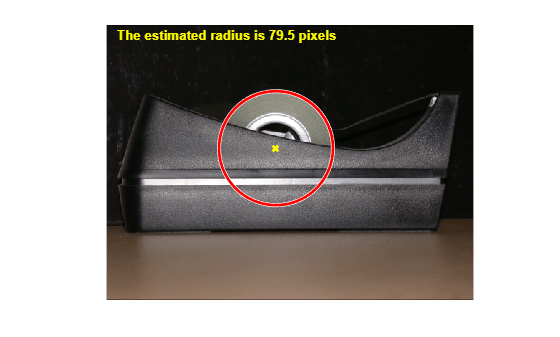Documentation

Measuring the Radius of a Roll of Tape

This example shows how to measure the radius of a roll of tape, which is partially obscured by the tape dispenser. Utilize imfindcircles to accomplish this task.

Step 1: Read Image

imshow(RGB);

hTxt = text(15,15,'Estimate radius of the roll of tape',...
'FontWeight','bold','Color','y');Step 2: Find the Circle

Find the center and the radius of the circle in the image using imfindcircles.

Rmin = 60;
Rmax = 100;
[center, radius] = imfindcircles(RGB,[Rmin Rmax],'Sensitivity',0.9)
center = 1×2

236.9291  172.4747

Step 3: Highlight the Circle Outline and Center

% Display the circle

% Display the calculated center
hold on;
plot(center(:,1),center(:,2),'yx','LineWidth',2);
hold off;

delete(hTxt);
message = sprintf('The estimated radius is %2.1f pixels', radius);
text(15,15,message,'Color','y','FontWeight','bold');